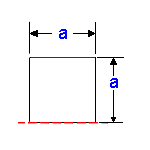Area Moment of Inertia Section Properties
Square Tube at Edge Calculator

Area Moment of Inertia Section Properties Square Tube at Edge Calculator and Equations

This engineering calculator will determine the section modulus and Area Moment of Inertia Section Properties for the given cross-section. This engineering data is often used in the design of structural beams or structural flexural members. There are two types of section moduli, the elastic section modulus (S) and the plastic section modulus (Z).

 Description Equation Area Moment of Inertia Section Properties = ISection Modulus = Z = I/yRadius of GyrationA = areay = distance from axis to extreme fiber y = aArea Moment of Inertia Section Properties Square Tube at Edge Calculator Inputs: Inch (in.) Metric (mm) "a"  (Side of Square) = Properties: Area Moment of Inertia Section Properties (Units4) = Section Modulus (Units3) = Radius of Gyration (Units) = Extreme Point (Units) = Area (Units2) =Membership Register | LoginHomeEngineering Book StoreEngineering ForumExcel App. DownloadsOnline Books & ManualsEngineering NewsEngineering VideosEngineering CalculatorsEngineering ToolboxGD&T Training Geometric Dimensioning TolerancingDFM DFA TrainingTraining Online EngineeringAdvertising CenterCopyright Notice